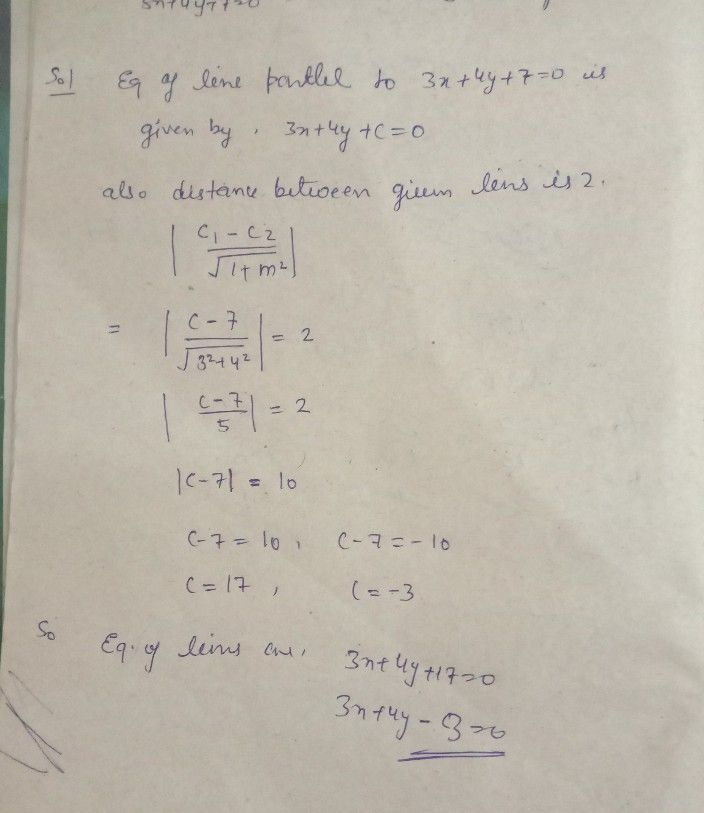Symbol
Problemaxes. ILs $201110$ 7. (a) Find the equation of the lines which is parallell to the line $3x+4y+7=0andisata$ distance 2 from it. $\left(b\right)$ Find the equations of the diagonals of the parallelogram formed by the lines $ax+by=0$ $ax+by+c=0,lx+my=0$ and $lx+my+n=0$ What is the condition that this $wi11be$ a rhombus ? (c) Find the equation of the line passing through the intersection $of2x-y-1=0and3x-$ $4y+6=.0$ and parallel to the line $x+y-2=0$ (d) Find the èquation of the line passing through the point of intersection of lines $x+3y+2=$ $andx-2y$ $4=0$ and perpendicular to the line $2y+5x-9=0$ $\left(e\right)$ Find the equation of the line passing through intersection of the lines.r+3y-1=0'and 3x $y+1=0$ $4\right)$ and the centroid ofthe triangle whose vertices are the points $s\left(3,-1\right)$ $\left(1.3\right)and\left(2$
10th-13th grade
Probability and Statistics
Search count: 109
SolutionQanda teacher - Aarjoo